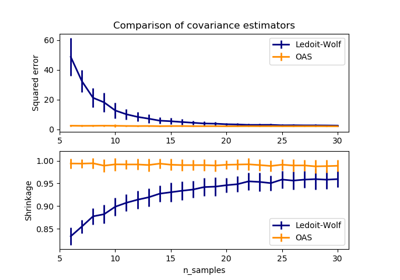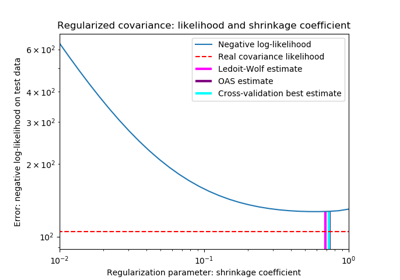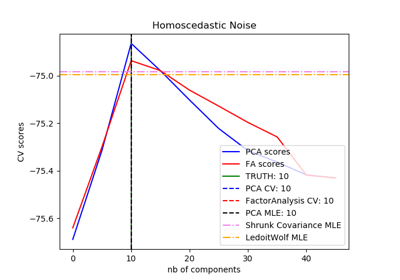# sklearn.covariance.LedoitWolf¶

class sklearn.covariance.LedoitWolf(store_precision=True, assume_centered=False, block_size=1000)[source]

LedoitWolf Estimator

Ledoit-Wolf is a particular form of shrinkage, where the shrinkage coefficient is computed using O. Ledoit and M. Wolf’s formula as described in “A Well-Conditioned Estimator for Large-Dimensional Covariance Matrices”, Ledoit and Wolf, Journal of Multivariate Analysis, Volume 88, Issue 2, February 2004, pages 365-411.

Read more in the User Guide.

Parameters: store_precision : bool, default=True Specify if the estimated precision is stored. assume_centered : bool, default=False If True, data are not centered before computation. Useful when working with data whose mean is almost, but not exactly zero. If False (default), data are centered before computation. block_size : int, default=1000 Size of the blocks into which the covariance matrix will be split during its Ledoit-Wolf estimation. This is purely a memory optimization and does not affect results. location_ : array-like, shape (n_features,) Estimated location, i.e. the estimated mean. covariance_ : array-like, shape (n_features, n_features) Estimated covariance matrix precision_ : array-like, shape (n_features, n_features) Estimated pseudo inverse matrix. (stored only if store_precision is True) shrinkage_ : float, 0 <= shrinkage <= 1 Coefficient in the convex combination used for the computation of the shrunk estimate.

Notes

The regularised covariance is:

(1 - shrinkage) * cov + shrinkage * mu * np.identity(n_features)

where mu = trace(cov) / n_features and shrinkage is given by the Ledoit and Wolf formula (see References)

References

“A Well-Conditioned Estimator for Large-Dimensional Covariance Matrices”, Ledoit and Wolf, Journal of Multivariate Analysis, Volume 88, Issue 2, February 2004, pages 365-411.

Examples

>>> import numpy as np
>>> from sklearn.covariance import LedoitWolf
>>> real_cov = np.array([[.4, .2],
...                      [.2, .8]])
>>> np.random.seed(0)
>>> X = np.random.multivariate_normal(mean=[0, 0],
...                                   cov=real_cov,
...                                   size=50)
>>> cov = LedoitWolf().fit(X)
>>> cov.covariance_
array([[0.4406..., 0.1616...],
[0.1616..., 0.8022...]])
>>> cov.location_
array([ 0.0595... , -0.0075...])


Methods

 error_norm(comp_cov[, norm, scaling, squared]) Computes the Mean Squared Error between two covariance estimators. fit(X[, y]) Fits the Ledoit-Wolf shrunk covariance model according to the given training data and parameters. get_params([deep]) Get parameters for this estimator. get_precision() Getter for the precision matrix. mahalanobis(X) Computes the squared Mahalanobis distances of given observations. score(X_test[, y]) Computes the log-likelihood of a Gaussian data set with self.covariance_ as an estimator of its covariance matrix. set_params(**params) Set the parameters of this estimator.
__init__(store_precision=True, assume_centered=False, block_size=1000)[source]
error_norm(comp_cov, norm='frobenius', scaling=True, squared=True)[source]

Computes the Mean Squared Error between two covariance estimators. (In the sense of the Frobenius norm).

Parameters: comp_cov : array-like, shape = [n_features, n_features] The covariance to compare with. norm : str The type of norm used to compute the error. Available error types: - ‘frobenius’ (default): sqrt(tr(A^t.A)) - ‘spectral’: sqrt(max(eigenvalues(A^t.A)) where A is the error (comp_cov - self.covariance_). scaling : bool If True (default), the squared error norm is divided by n_features. If False, the squared error norm is not rescaled. squared : bool Whether to compute the squared error norm or the error norm. If True (default), the squared error norm is returned. If False, the error norm is returned. The Mean Squared Error (in the sense of the Frobenius norm) between self and comp_cov covariance estimators.
fit(X, y=None)[source]

Fits the Ledoit-Wolf shrunk covariance model according to the given training data and parameters.

Parameters: X : array-like, shape = [n_samples, n_features] Training data, where n_samples is the number of samples and n_features is the number of features. y not used, present for API consistence purpose. self : object
get_params(deep=True)[source]

Get parameters for this estimator.

Parameters: deep : boolean, optional If True, will return the parameters for this estimator and contained subobjects that are estimators. params : mapping of string to any Parameter names mapped to their values.
get_precision()[source]

Getter for the precision matrix.

Returns: precision_ : array-like The precision matrix associated to the current covariance object.
mahalanobis(X)[source]

Computes the squared Mahalanobis distances of given observations.

Parameters: X : array-like, shape = [n_samples, n_features] The observations, the Mahalanobis distances of the which we compute. Observations are assumed to be drawn from the same distribution than the data used in fit. dist : array, shape = [n_samples,] Squared Mahalanobis distances of the observations.
score(X_test, y=None)[source]

Computes the log-likelihood of a Gaussian data set with self.covariance_ as an estimator of its covariance matrix.

Parameters: X_test : array-like, shape = [n_samples, n_features] Test data of which we compute the likelihood, where n_samples is the number of samples and n_features is the number of features. X_test is assumed to be drawn from the same distribution than the data used in fit (including centering). y not used, present for API consistence purpose. res : float The likelihood of the data set with self.covariance_ as an estimator of its covariance matrix.
set_params(**params)[source]

Set the parameters of this estimator.

The method works on simple estimators as well as on nested objects (such as pipelines). The latter have parameters of the form <component>__<parameter> so that it’s possible to update each component of a nested object.

Returns: self

## Examples using sklearn.covariance.LedoitWolf¶Ledoit-Wolf vs OAS estimationShrinkage covariance estimation: LedoitWolf vs OAS and max-likelihoodModel selection with Probabilistic PCA and Factor Analysis (FA)